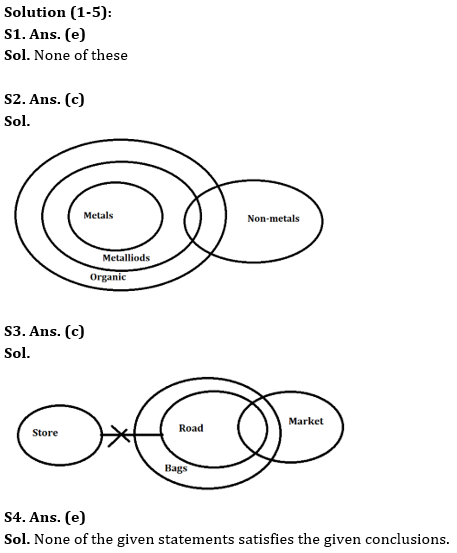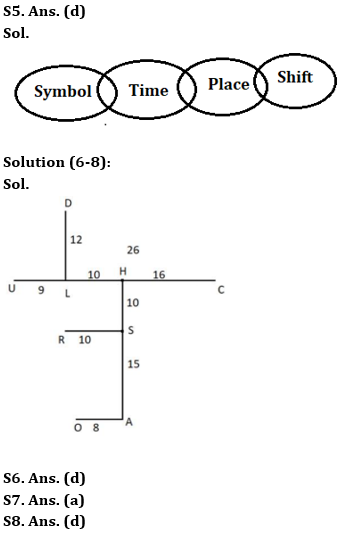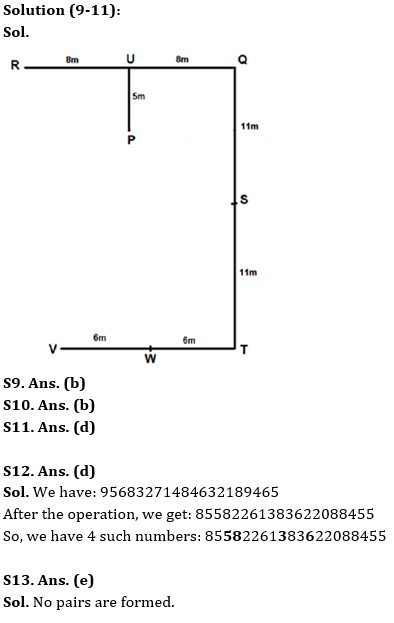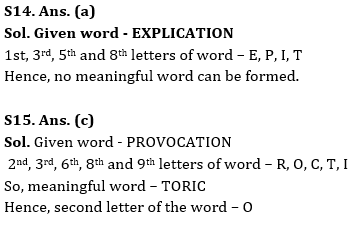Latest Banking jobs   »   Reasoning Ability Quiz For SBI PO/Clerk...

# Reasoning Ability Quiz For SBI PO/Clerk Mains 2023- 5th January

Directions (1-5): In the question below there are two conclusions followed by three statements in the options. You have to take the given three statements to be true even if they seem to be at variance from the commonly known facts and then decide which of the given statements are true disregarding the commonly known facts.

Q1. Conclusions: Few Bags are not Levi’s. Some Pepe are Belts.
(a) Only a few Bags are Belts. All Levi’s are Belts. Few Pepe are Levi’s.
(b) Only Levi’s are Belts. Some Levi’s are Pepe. All Bags are Pepe.
(c) Only a few Pepe are Levi’s. All Bags are Belts. Some Belts are Levi’s.
(d) All Bags are Belts. All Belts are Pepe. All Pepe are Levi’s.
(e) None of the above

Q2. Conclusions: Some Non-metals are Organic. All Metals can be Non-metals.
(a) Some Metals are Organic. All non-metals are Metalloids. No Organic are Metalloids.
(b) Some Metalloids are Organic. No Metalloids are Metals. All non-metals are Metals.
(c) All Metals are Metalloids. Some Non-metals are Metalloids. All Metalloids are Organic.
(d) Only a few Non-metals are Metalloids. All Organic are Metals. Few Organic are Metalloids.
(e) Both option (b) and option (d) are correct.

Q3. Conclusions: All bags can never be markets. Some markets being stores is a possibility.
(a) Only a few bears are bags. No bear is a store. All stores are markets.
(b) Some markets are bags. Only a few bags are stores. Only a few markets are chores.
(d) All chores are stores. Only a few chores are bags. Some markets are not bags.
(e) None of these

Q4. Conclusions: At least some rodents are pests. Some pests are definitely not coins.
(a) Only a few mice are pests. Some mice are coins. All coins are pages. Some pages are rodents.
(b) Only a few prizes are rodents. All rodents are snakes. No snake is a pest. All pests are coins.
(c) Only a few pages are pests. Some pests are coins. Only a few coins are rodents. No rodent is a page.
(d) Only a few rats are rodents. All rodents are coins. Some coins are rats. Some pests are rats.
(e) None of these

Q5. Conclusions: Some symbol not being shift is a possibility. All time can never be place.
(a) All shift is time. Some Time is place. Some symbol is not place.
(b) Some place is not time. Some time is shift. Only shift is symbol.
(c) All symbol are place. All place are shift. No symbol is time.
(d) Some symbol is time. Only a few time is place. Some place is shift.
(e) None of these

Directions (6-8): The following are the codes used for denoting the directions between two points:
M? (40) N means M is 4 m to the north of N
M*(91) N means M is 10 m to the east of N
M %(467) N means M is 17 m to the south of N
M = (23) N means M is 5 m to the west of N
M?* (158) N means M is 14 m to the north-east of N

The distance and directions between some points are given below:
L= (899) C, H*(946) U, A%(979) H, R= (55) S, A*(80) O, H? (37) S, D? (66) L, H= (88) C

Q6. Which of the following is correct?
I. S?* (87) O
II. U%= (78) D
III. R% (64) D
(a) Only II and III
(b) Only I
(c) Only I and III
(d) Only II
(e) Only III

Q7. Which of the following points are in a straight vertical line?
(a) DR
(b) OL
(c) CA
(d) UL
(e) OC

Q8. Which of the following point is the nearest to point R?
(a) O
(b) U
(c) H
(d) L
(e) A

Directions (9-11): Answer the questions based on the information given below.
X% (5m) Y – X is 5m north of Y.
X # (6m) Y – X is 6m south of Y.
X @ (8m) Y – X is 8m east of Y.
X \$ (7m) Y – X is 7m west of Y.
X * YZ – X is midpoint of vertical straight line YZ.
X & YZ – X is midpoint of horizontal straight line YZ.

A certain number of people are sitting in a park at some distance.
U % (5m) P, U & RQ, R \$ (8m) U, T # (11m) S, S*QT, V \$ (12)T, W & VT

Q9. What is the direction of P with respect to W?
(a) North-east
(b) North-west
(c) South-west
(d) South-east
(e) None of these

Q10. What is the direction of R with respect to T?
(a) North-east
(b) North-west
(c) South-west
(d) South-east
(e) None of these

Q11. If “P \$ (8m) X”, then what is the shortest distance between X and S?
(a) 3m
(b) 4m
(c) 5m
(d) 6m
(e) None of these

Q12. If in the number “95683271484632189465”, the number at each odd position is decreased by 1, then how many such numbers are there in the final arrangement each of which is immediately preceded by an odd number and immediately followed by an even number?
(a) 2
(b) 5
(c) 3
(d) 4
(e) More than 5

Q13. How many pairs of letters are there in the word ‘SAGACITY’ which has as many letters between them in English alphabetical series (both in forward and backward direction)?
(a) Three
(b) Four
(c) Five
(d) Two
(e) None

Q14. If it is possible to make a meaningful word from 1st, 3rd, 5th and 8th letters of word “EXPLICATION” (using each letter once), then which will be the 3rd letter of the word? If no such word be formed, mark the answer as ‘X’. If more than one word can be formed mark the answer as ‘Y’.
(a) X
(b) E
(c) I
(d) P
(e) Y

Q15. If it is possible to make a meaningful word from 2nd, 3rd, 6th, 8th and 9th letters of word “PROVOCATION”(using each letter once), then which will be the 2nd letter of the word? If no such word be formed, mark the answer as ‘X’. If more than one word can be formed mark the answer as ‘Y’.
(a) X
(b) T
(c) O
(d) R
(e) Y

Solutions## FAQs

### Q1. Conclusions: Few Bags are not Levi's. Some Pepe are Belts.

S1. Ans. (e)
Sol. None of these

#### Congratulations!